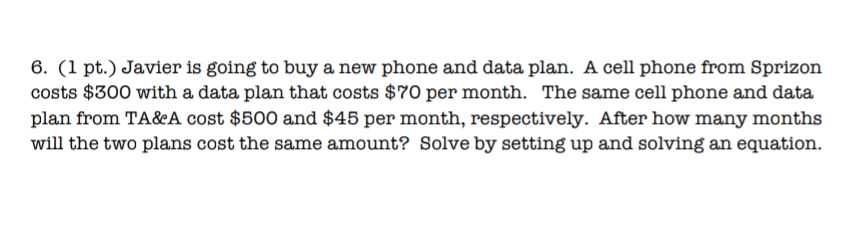### ¿Todavía tienes preguntas de matemáticas?

Pregunte a nuestros tutores expertos
Algebra
Pregunta6. (1 pt.) Javier is going to buy a new phone and data plan. A cell phone from Sprizon costs $$\ 300$$ with a data plan that costs $$\ 70$$ per month. The same cell phone and data plan from TA\&eA cost $$\ 500$$ and $$\ 45$$ per month, respectively. After how many months will the two plans cost the same amount? Solve by setting up and solving an equation.

$$300+ 70x= 500+ 45x\\25x= 200\\x= 8$$